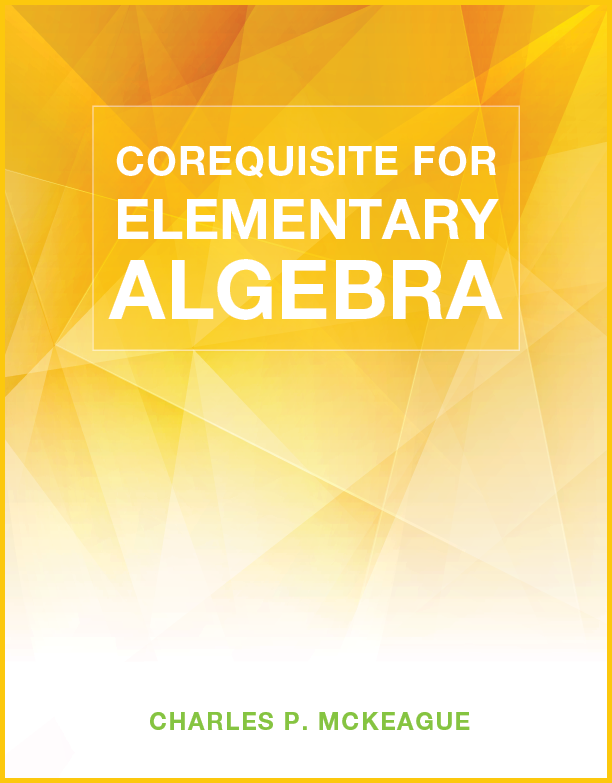## Corequisite for Elementary Algebra

### Author: Charles P. McKeagueLessons:

Lesson 1: Positive and Negative Numbers
Lesson 2: Addition with Negative Numbers
Lesson 3: Subtraction with Negative Numbers
Lesson 4: Multiplication with Negative Numbers
Lesson 5: Division with Negative Numbers
Lesson 6: Simplifying Algebraic Expressions
Lesson 7: Prime Numbers, Factors, and Reducing to Lowest Terms
Lesson 8: Multiplication with Fractions, and The Area of a Triangle
Lesson 9: The Distributive Property and Algebraic Expressions
Lesson 10: The Addition Property of Equality
Lesson 11: The Multiplication Property of Equality
Lesson 12: Linear Equations in One Variable
Lesson 13: Fractions and Decimals
Lesson 14: Equations Containing Decimals
Lesson 15: Square Roots and the Pythagorean Theorem
Lesson 16: Proportions
Lesson 17: Applications of Proportions
Lesson 18: Percents, Decimals, and Fractions
Lesson 19: Basic Percent Problems
Lesson 20: Paired Data and Graphing Ordered Pairs